### Home > CAAC > Chapter 8 > Lesson 8.1.2 > Problem8-17

8-17.
1. For each rule represented below, state the x- and y-intercepts, if possible. Homework Help ✎

1.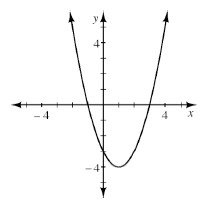2.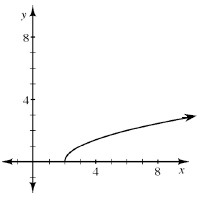3.  x −5 −4 −3 −2 −1 0 1 2 y 8 4 0 −4 0 2 0 −4
4. 5x − 2y = 40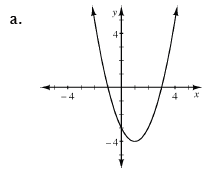The x-intercept is where y = 0.
The y-intercept is where x = 0.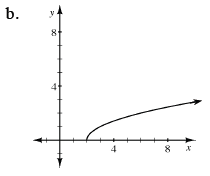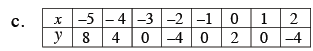Look for the zeros in the table.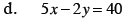The y-intercept is (0, y) and the x-intercept is (x, 0).